# Eccentricity

(diff) ← Older revision | Latest revision (diff) | Newer revision → (diff)
The number equal to the ratio of the distance between any point of a conic section (cf. Conic sections) and a given point (the focus) to that between the same point and a given line (the directrix). Two conic sections having the same eccentricity are similar. For an ellipse the eccentricity is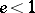(for a circle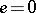), for a hyperbola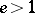, and for a parabola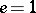. For an ellipse and hyperbola the eccentricity can also be defined as the ratio of the distances between the foci and the length of the major axis.
For the "standard" ellipse, parabola and hyperbola, given, respectively, by the equations,, and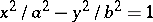, the eccentricity is equal to, respectively,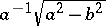(if), 1 and. A focusand a corresponding directrixfor the three cases are given by,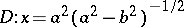(if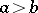);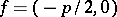,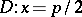;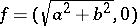,. There are two foci for ellipses and hyperbolas and there is one for parabolas.